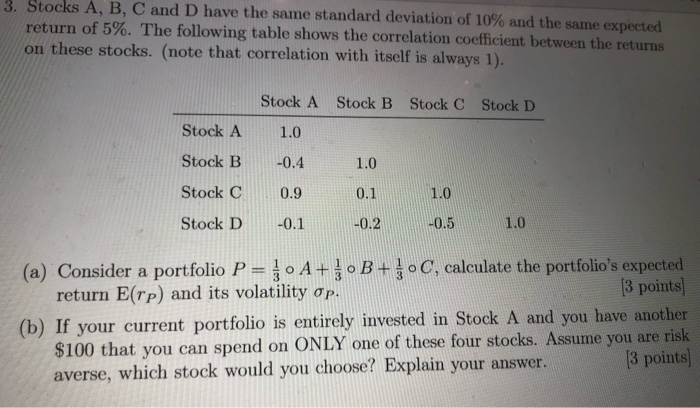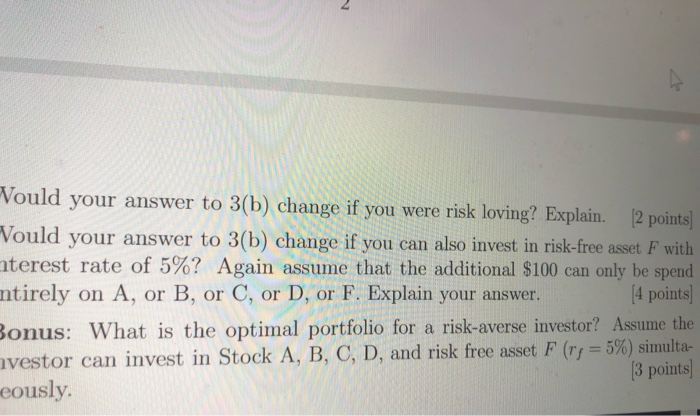### Create an Account

Already have account?

### Forgot Your Password ?

Home / Questions / Stocks A B C and D have the same standard deviation of 10% and the same expected return o...

# Stocks A B C and D have the same standard deviation of 10% and the same expected return of 5% The following table shows Vould your answer to 3 change if you were risk loving3. Stocks A, B, C and D have the same standard deviation of 10% and the same expected return of 5%. The following table shows the correlation coefficient between the returns on these stocks. (note that correlation with itself is always 1). Stock B Stock C Stock D Stock A Stock B Stock C Stock D Stock A 1.0 -0.4 0.9 -0.1 1.0 0.1 1.0 -0.5 -0.2 1.0 (a) Consider a portfolio P = 0 A+ B+ C, calculate the portfolio&#39;s expected return E(rp) and its volatility op. (3 points) (b) If your current portfolio is entirely invested in Stock A and you have another \$100 that you can spend on ONLY one of these four stocks. Assume you are risk averse, which stock would you choose? Explain your answer. [3 points) Vould your answer to 3(b) change if you were risk loving? Explain. [2 points) Vould your answer to 3(b) change if you can also invest in risk-free asset F with nterest rate of 5%? Again assume that the additional \$100 can only be spend ntirely on A, or B, or C, or D, or F. Explain your answer. (4 points) Bonus: What is the optimal portfolio for a risk-averse investor? Assume the nvestor can invest in Stock A, B, C, D, and risk free asset F (ry = 5%) simulta- 3 points) eously.

Feb 08 2020 View more View Less

#### Answer (UnSolved)Get Solution